# Top Statistics Formulas

Here you will find the most common statistics equations and formulas used in high school and fundamental university courses. These include basic statistical measures and probability formulas. For more calculus based statistics formulas, please see here.

## Basic Equations

### Average or Mean

Where xi are all the individual data points, and N is the number of points being averaged

=
 xi N
=
 sum of observations # of observations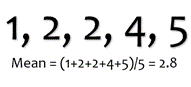### Median

The middle value in the set of numbers that are arranged in ascending order. If there are an even number of values, the median is the average of the two middle values.

If n is odd        (
 n+1 2
) th term
If n is even      (
 n 2
) th term + (
 n 2
+ 1 ) th term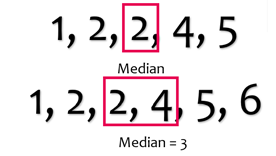### Mode

The most frequently occurring value in the set of values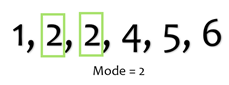### Percent Change

+ Means an increase
- Means a decrease

% Change =
 New Value - Old Value Old Value
×100%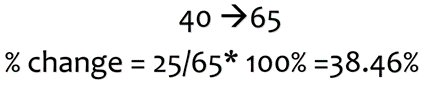## Statistical Measures

### Standard Deviation

Standard Deviation is one measure of how spread the data is.

Sample      S =  √
 ∑ (xi - x̄ )2 n-1

Where x̄ is the sample mean and n is the number of observations

Population       σ =  √
 ∑ (xi - μ̄ )2 n

Where μ̄ is the population mean and n is the number of observations

### Variance

Variance is one measure of how spread the data is. It is the standard deviation squared.

Sample      S 2 =
 ∑(xi - x̄ )2 n-1

Where x̄ is the sample mean and n is the number of observations

Population       σ 2 =
 ∑(xi - μ̄ )2 n

Where μ̄ is the population mean and n is the number of observations

### Covariance

Covariance is a measure of two variables’ joint variability, or how they will vary together.

Sample      Cov(x,y) =
 ∑(xi - x̄ ) * (yi - ȳ ) n-1

The measure of variance between variables x and y, where x̄ is the sample mean of the x values, ȳ is the mean of the y values, and n is the number of observations

Population       Cov(x,y) =
 ∑(xi - x̄ ) * (yi - ȳ ) n

### Chi Squared

Chi Squared tells you the difference between your observations and what you expected.

X2 =
 (Oi - Ei )2 Ei

Where Oi is the observed value and Ei is the expected value

## Probability

### Probability of Event A

P(A) =
 f n

Where f is the frequency of the event and n is the sample size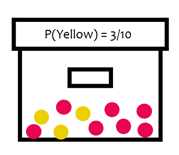### Probability of Complement of Event A

In other words, the probability of A not occurring.

 P(Not A) = 1 - P(A)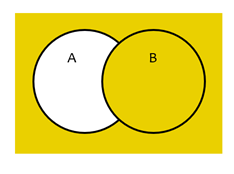### Multiplication Rule Independent Events

In other words, the probability of A and B occurring independently.

 P(A and B) = P(A) * P(B)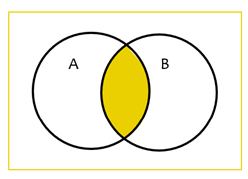### General Multiplication Rules

To be used when one event A affects the probability of event B.

 P(A and B) = P(A) * P(B given A) P(A and B) = P(B) * P(A given B)

For example,
Drawing marbles out of the box without replacement will affect the probability of drawing a marble of a certain color.

### Addition Rule Mutually Exclusive Events

In other words, the probability of either A or B occurring.

 P(A or B) = P(A) + P(B)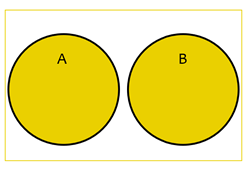In other words, the probability of either A or B occurring.

 P(A or B) = P(A) + P(B) - P(A and B)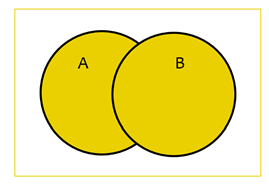### Expected Value

Expected Value is the final predicted value of a variable, computed by multiplying each possible value by its probability and summing it.

 E[x] = ∑ xiP ( xi )

Where xi are the values of x and P(xi) are the probabilities of each value